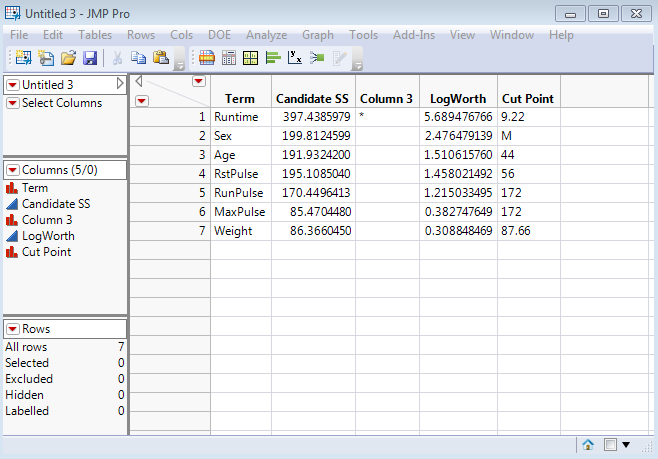Choose Language Hide Translation Bar
Highlighted

## Partition analysis JSL - Candidates, make into data table

Hi Folks,

Im just wondering if there is anyway to write a script that pulls out the split candidates from a partition analysis in a data table, ordered by logworth. This can be done manually when you fit a partition model and right click on the candidates section.

1 ACCEPTED SOLUTION

Accepted SolutionsSuper UserJoined:

Jul 13, 2011

Solution

## Re: Partition analysis JSL - Candidates, make into data table

Scott

I do this quite a lot.  Here is anexample of  the code that I use

( the answer to your question are the 4 lines in the section "extract the candidates table" ! )

// sample data

dt = Open("\$SAMPLE_DATA/Fitness.jmp");

dtName = dt << Get Name;

// select response variable

colNames = dt << Get Column Names(string);

nw = New Window("Select Y", <<Modal,

lb = List Box(colNames, MaxSelected(1), <<Set Selected(5))

);

If (nw["Button"]==-1, Throw());

sel = lb << Get Selected;

If (Nitems(sel)==0,

Throw()

,

yCol = sel

);

// use all other columns as x variables

pos = Loc(colNames,yCol);

xCols = Remove(colNames,pos);

// drop name column

RemoveFrom(xCols,1);

// launch the partition platform

pat = Partition(

Y( Eval(yCol) ),

X( Eval(xCols) ),

);

// extract the candidates table

rep = pat << Report;

candTab = rep["Candidates"][TableBox(1)];

candDt = candTab << Make Into Data Table;

rep << Close Window;

// sort the candidates table

candDt << Sort( By( :LogWorth ), Order( Descending ), Replace Table );

// icing on the cake - append a script so that row selections

// in this table will generate column selections in the source table

Eval(Parse(Eval Insert("\[

candDt << New Script(

"Select Columns",

cdt = Current Data Table();

rows = cdt << Get Selected Rows;

lstNames = Column(cdt,"Term")[rows];

Data Table("^dtName^") << Clear Column Selection;

For (i=1,i<=NItems(lstNames),i++,

Column( Data Table("^dtName^"), lstNames ) << Set Selected(1)

)

)

]\")));

The end result is a table that looks like this:I included the code for the Select Columns script attached to this table as I find it particularly useful.  So for example if you select the first three rows on this candidates table then running the script will select the corresponding columns in the source data table.  Useful if you have loads of columns.

- Dave

-Dave
2 REPLIES 2Super UserJoined:

Jul 13, 2011

Solution

## Re: Partition analysis JSL - Candidates, make into data table

Scott

I do this quite a lot.  Here is anexample of  the code that I use

( the answer to your question are the 4 lines in the section "extract the candidates table" ! )

// sample data

dt = Open("\$SAMPLE_DATA/Fitness.jmp");

dtName = dt << Get Name;

// select response variable

colNames = dt << Get Column Names(string);

nw = New Window("Select Y", <<Modal,

lb = List Box(colNames, MaxSelected(1), <<Set Selected(5))

);

If (nw["Button"]==-1, Throw());

sel = lb << Get Selected;

If (Nitems(sel)==0,

Throw()

,

yCol = sel

);

// use all other columns as x variables

pos = Loc(colNames,yCol);

xCols = Remove(colNames,pos);

// drop name column

RemoveFrom(xCols,1);

// launch the partition platform

pat = Partition(

Y( Eval(yCol) ),

X( Eval(xCols) ),

);

// extract the candidates table

rep = pat << Report;

candTab = rep["Candidates"][TableBox(1)];

candDt = candTab << Make Into Data Table;

rep << Close Window;

// sort the candidates table

candDt << Sort( By( :LogWorth ), Order( Descending ), Replace Table );

// icing on the cake - append a script so that row selections

// in this table will generate column selections in the source table

Eval(Parse(Eval Insert("\[

candDt << New Script(

"Select Columns",

cdt = Current Data Table();

rows = cdt << Get Selected Rows;

lstNames = Column(cdt,"Term")[rows];

Data Table("^dtName^") << Clear Column Selection;

For (i=1,i<=NItems(lstNames),i++,

Column( Data Table("^dtName^"), lstNames ) << Set Selected(1)

)

)

]\")));

The end result is a table that looks like this:I included the code for the Select Columns script attached to this table as I find it particularly useful.  So for example if you select the first three rows on this candidates table then running the script will select the corresponding columns in the source data table.  Useful if you have loads of columns.

- Dave

-Dave

## Re: Partition analysis JSL - Candidates, make into data table

Perfect David, just what I was looking for